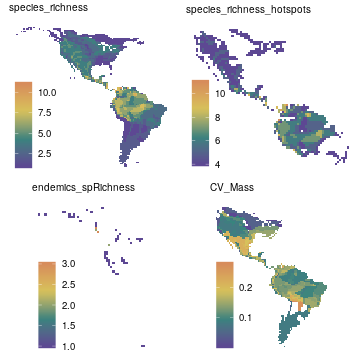## Appendix S2

Supporting information in Valcu, M., Dale, J., and Kempenaers, B. (2012). rangeMapper: a platform for the study of macroecology of life-history traits. Global Ecology and Biogeography 21, 945-951.

The example shown here is run on the wrens dataset which is part of the package. The wrens dataset has 84 species while the case study presented in the paper was run on 8434 bird species. Therefore both the settings and the results shown below are not identical with the results presented in Valcu et al 2012.

### Project Set Up

Initiates a new rangeMapper project (`wrens.sqlite`) into a temporary directory

``````td = tempdir()
require(rangeMapper)
``````
``````## Loading required package: rangeMapper
``````
``````## Loading required package: RSQLite
``````
``````## This is rangeMapper 0.3-7
``````
``````dbcon = rangeMap.start(file = "wrens.sqlite", dir = td, overwrite = TRUE)
``````
``````## New session 2019-10-25 17:52:20
``````
``````## PROJECT: wrens.sqlite
## DIRECTORY: /tmp/Rtmpe4D7pf
``````
``````# Location of the vector(*.shp) breeding ranges on disk
branges = system.file(package = "rangeMapper", "extdata", "wrens", "vector_combined")
``````

Saves the global bounding box as the union of all species bounding boxes.

``````global.bbox.save(con = dbcon, bbox = branges)
``````
``````## Computing global bounding box for 1 ranges...
``````
``````## Checking for proj4 string differences...
``````
``````## Done!
``````
``````##  TRUE
``````

Saves the grid size (i.e. the size of a canvas cell) using the default value. The grid size can be specified by e.g. gridSize.save(dbcon, gridSize = 2.5)

``````gridSize.save(dbcon)
``````
``````## Warning in .local(object, ...): Default grid size used!
``````
``````## Grid size set to 1.1728375626 map units.
``````

Saves the canvas grid using the global bounding box and the grid size.

``````canvas.save(dbcon)
``````
``````## Canvas uploaded.
``````

Performs vector range maps interpolation with the canvas.

``````r = rgdal::readOGR(branges, "wrens", verbose = FALSE)
processRanges(spdf = r, con =  dbcon, ID = "sci_name", metadata = rangeTraits() )
``````
``````## Warning: executing %dopar% sequentially: no parallel backend registered
``````
``````## Writing overlay output to project...
``````
``````## 84 out of 84 ranges updated; Elapsed time: 0 mins
``````
``````## Extracting metadata...
``````

Uploads to the existing project a `data.frame` containing life history data

``````data(wrens)
bio.save(con = dbcon, loc = wrens, ID = "sci_name")
``````
``````## Table wrens saved as a  BIO_ table
``````

### Define Hotspots Parameters

(i.e. the quantile of the given parameter used as a threshold value)

``````P_richness = 0.75 # species richness probability
P_endemics = 0.35 # endemic species richness probability
``````

#### Hotspots Of Species Richness Map

``````# 1) Save the species richness (SR) map
rangeMap.save(dbcon, tableName = "species_richness")
``````
``````##  TRUE
``````
``````# 2) Fetch the SR map and find the SR value corresponding with the probability
# previously defined by P_richness
sr = rangeMap.fetch(dbcon, "species_richness")
sr_threshold = quantile(sr\$species_richness, probs = P_richness, na.rm = TRUE)

# 3) Save the SR hotspots map; only canvas cells with species_richness >= sr_threshold will be included
rangeMap.save(dbcon, tableName = "species_richness_hotspots",
subset = list(MAP_species_richness =
paste("species_richness >=", sr_threshold)) )
``````
``````##  TRUE
``````

#### Hotspots Of Endemic Species Map

``````# 1) Save endemics species richness map
es = dbGetQuery(dbcon, "select Area from metadata_ranges")
es_threshold = quantile(es\$Area, probs = P_endemics, na.rm = TRUE)

rangeMap.save(dbcon, tableName = "endemics_spRichness",subset =
list(metadata_ranges = paste("Area <= ", es_threshold) ) )
``````
``````##  TRUE
``````
``````# 2) Save hotspots of endemics
er = rangeMap.fetch(dbcon, "endemics_spRichness")
er_threshold = quantile(er\$endemics_spRichness, probs = P_richness, na.rm = TRUE)

rangeMap.save(dbcon, tableName = "endemics_hotspots", subset = list(
MAP_endemics_spRichness = paste("endemics_spRichness >=", er_threshold) ) )
``````
``````##  TRUE
``````

#### Hotspots Of Body Mass Map

``````#1) Save Coefficient of variation (CV_Mass) map using a function (FUN) defined
# on the fly. The ... argument of the function will allow further arguments
# in this case 'na.rm = TRUE' to be passed to FUN.

rangeMap.save(dbcon,
FUN = function(x,...) (sd(log(x),...)/mean(log(x),...)),
na.rm = TRUE, biotab = "wrens", biotrait = "body_mass",
tableName = "CV_Mass")
``````
``````##  TRUE
``````
``````# 2) Find the 'CV_Mass' threshold which corresponds with 'P_richness'
bmr = rangeMap.fetch(dbcon, "CV_Mass")
bmr_threshold = quantile(bmr\$CV_Mass, probs = P_richness, na.rm = TRUE)

# 3) Save bodyMass richness hotspots map using the computed threshold
rangeMap.save(dbcon, tableName = "bodyMass_richness_hotspots",
subset = list(MAP_CV_Mass =
paste("body_mass >=", bmr_threshold) ) )
``````
``````##  TRUE
``````
``````#  FETCH MAPS & PLOT
SRmaps = rangeMap.fetch(dbcon, c("species_richness", "species_richness_hotspots",
"endemics_spRichness", "CV_Mass"), spatial = FALSE )
plot(SRmaps)
``````### HOTSPOTS CONGRUENCE

1) Find threshold values

``````sr = rangeMap.fetch(dbcon, "species_richness")
Q_richnes = quantile(sr\$species_richness, probs = P_richness, na.rm = TRUE)

er = rangeMap.fetch(dbcon, "endemics_spRichness")
Q_endemics = quantile(er\$endemics_spRichness, probs = P_richness, na.rm = TRUE)

bmr = rangeMap.fetch(dbcon, "CV_Mass")
Q_bodyMass = quantile(bmr\$CV_Mass, probs = P_richness,na.rm = TRUE)
``````

2) Save cumulative hotspot congruence satisfying all three criteria

``````rangeMap.save(dbcon, tableName = "cumul_congruence_hotspots",subset = list(
MAP_CV_Mass             = paste("body_mass>=",Q_bodyMass),
MAP_species_richness    = paste("species_richness>=",Q_richnes),
MAP_endemics_spRichness = paste("endemics_spRichness>=",Q_endemics) ))
``````
``````##  TRUE
``````

3) Species richness & endemics richness congruence (two criteria are satified)

``````rangeMap.save(dbcon, tableName = "SR_ER_congruence_hotspots",subset = list(
MAP_species_richness    = paste("species_richness>=",Q_richnes),
MAP_endemics_spRichness = paste("endemics_spRichness>=",Q_endemics) ))
``````
``````##  TRUE
``````

4) Endemics richness & body mass diversity congruence

``````rangeMap.save(dbcon, tableName = "ER_BR_congruence_hotspots",
subset = list(
MAP_CV_Mass=paste("body_mass>=",Q_bodyMass),
MAP_endemics_spRichness=paste("endemics_spRichness>=",Q_endemics) ))
``````
``````##  TRUE
``````

5) Species richness & body mass diversity congruence

``````rangeMap.save(dbcon, tableName = "SR_BR_congruence_hotspots",
subset = list(
MAP_CV_Mass=paste("body_mass>=",Q_bodyMass),
MAP_species_richness=paste("species_richness>=",Q_richnes) ))
``````
``````##  TRUE
``````

6) Retrieve all the map objects.

``````m = rangeMap.fetch(dbcon, c("species_richness_hotspots","endemics_hotspots",
"bodyMass_richness_hotspots","cumul_congruence_hotspots",
"SR_ER_congruence_hotspots","SR_BR_congruence_hotspots",
"ER_BR_congruence_hotspots") )
``````

7) Compute hotspots congruence

``````# The data slot of the map 'm' can be queried for presence/absence using the 'is.na' function.

sr = length( which(!is.na(m\$species_richness_hotspots)))
er = length( which(!is.na(m\$endemics_hotspots)))
br = length( which(!is.na(m\$bodyMass_richness_hotspots)))

srer = length( which(!is.na(na.omit(m\$SR_ER_congruence_hotspots))) )
srbr = length( which(!is.na(na.omit(m\$SR_BR_congruence_hotspots))) )
erbr = length( which(!is.na(na.omit(m\$ER_BR_congruence_hotspots))) )

cum = length( which(!is.na(na.omit(m\$cumul_congruence_hotspots))) )
``````

Species richness & body mass diversity congruence (%)

``````(srbr/(sr+br))*100
``````
``````##  18.35821
``````

Endemics richness & body mass diversity congruence (%)

``````(erbr/(er+br))*100
``````
``````##  5.306122
``````

Species richness & endemics richness congruence (%)

``````(srer/(sr+er))*100
``````
``````##  4.583333
``````

Cumulative congruence (%)

``````(cum/(sr+er+br))*100
``````
``````##  1.648746
``````